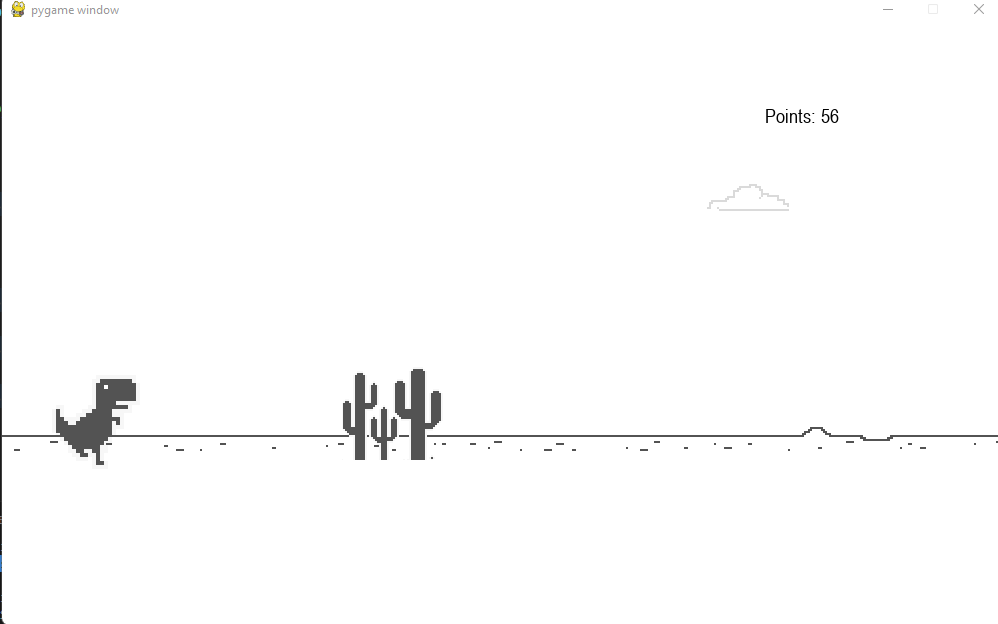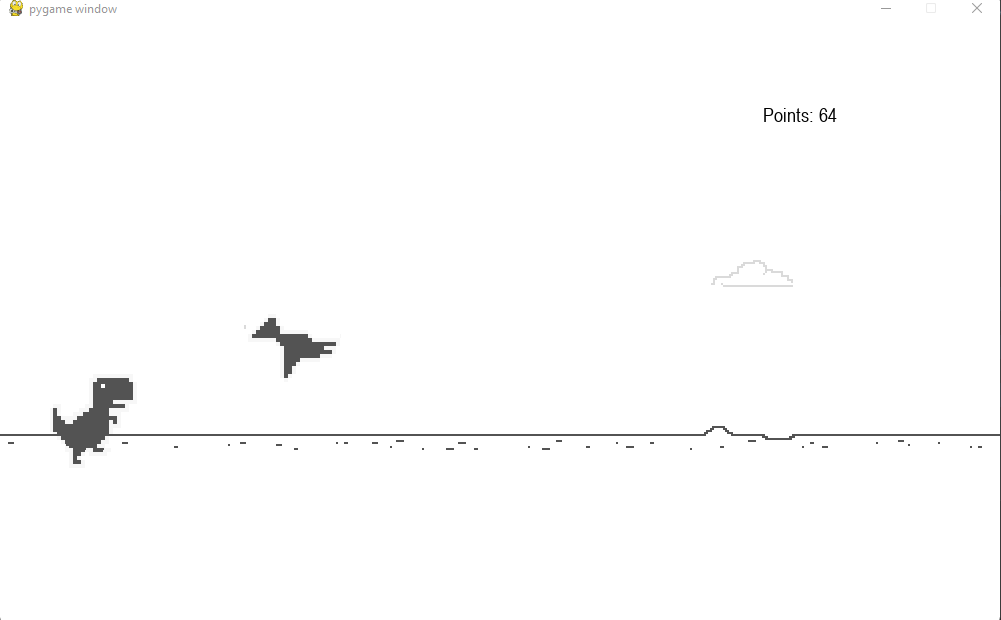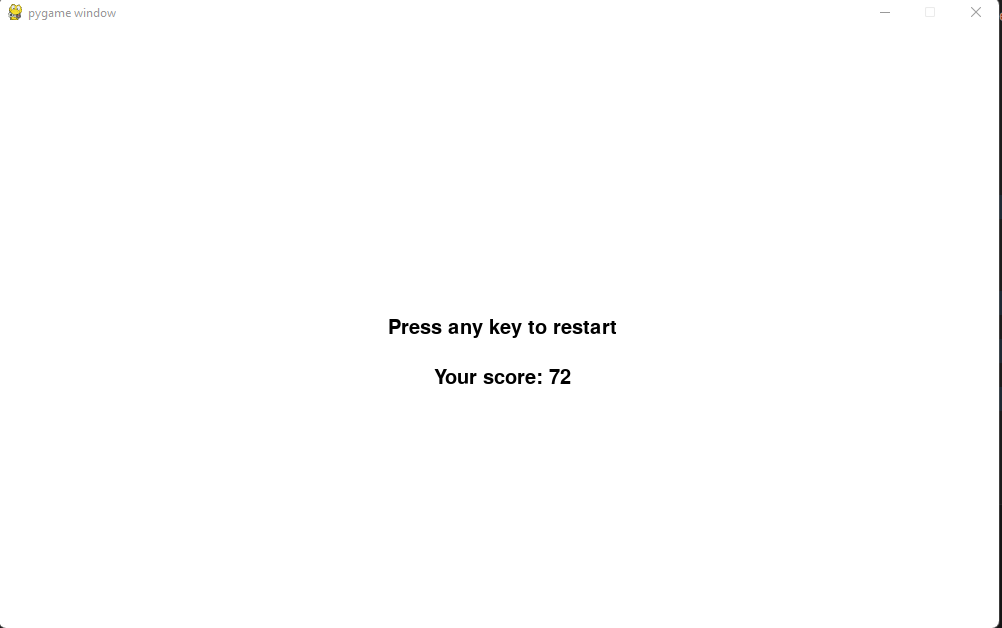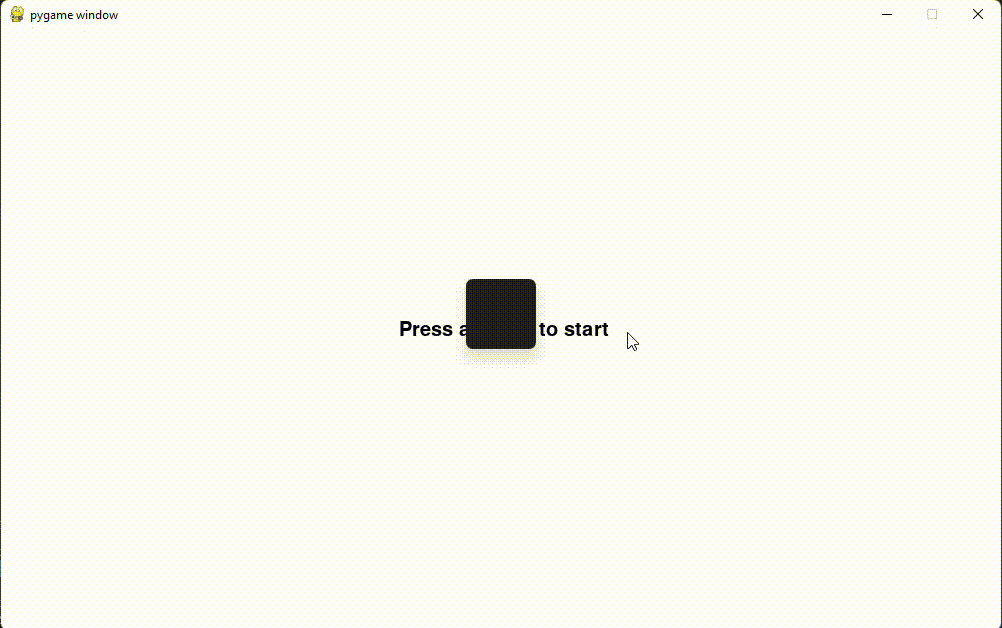×

Search anything:

# Chrome dinosaur game in Python

#### PythonGet this book -> Problems on Array: For Interviews and Competitive Programming

In this article at OpenGenus, we will be looking at how to make the chrome dinosaur game in Python Programming Language.

The flow of the article is:

• A brief introduction to pygame and story telling.
• Implementation
• Requirements.
• Aim. (Where are we trying to get?)
• Code Structure.
• Creating Dinosaur class.
• Creating Cloud class
• Creating Main.py
• Final Result

### Introduction:

To make a GUI based game, we will use the module pygame which is used to make a lot of games using python. pygame has graphic and sound libraries which help aid the process of game development.

We will be going over 3 main steps in order to make a game. Firstly, principle idea. Secondly, story. Thirdly, implementation. A video game is another form of story telling, it is attracts a lot of user attention and enslaves them. It's addictive and entertaining both.

Principle Idea: This is the stage where the general idea of why a game is to be made and the basic theme of it should be thought of. In our case, we are going to make a game which user can play when they are not connected to the network.

Story: The story is an essential component which drives the gamer. The story in our game is going to be simple, run and get through the obstacles. The more the gamer runs, the faster the gamespeed will become.

### Requirements of the game:

1.Python provides us with a library which can be used for game development.
We are going to use pygame library in our game. The way to install pygame onto our system is by using pip which is the python's package installation manager.

``````pip install pygame
``````
1. To create the game, we are in need of different classes which cater different needs. The primary classes in play are dinosaur class(this is the user or the dinosaur which is running, jumping or ducking on screen).
2. The background of the game consists of clouds which is created using cloud class.
3. The obstacles that the user encounters on their journey should be created. This can be done using obstacle class.
4. The obstacle class is an important class in the context of this game because several necessary classes like small cactus, large cactus and the bird take some important characteristics from the base class i.e obstacle class.
5. Other than these classes, there are several important functions which come into play such as a function which takes care of score, blits out a track for the user to run on, creates menus for operational management using inputs from the user of the game.

### What we are trying to get to:Dino with a cactus:Dino with a bird:### Code Structure:

We are going to structure our code in the following way.

```-Dino_game
+ Assets
- main.py
- dinosaur.py
- cloud.py
```

The assets folder contains images which aid in making animations and background for our game.

### Creating Dinosaur Class.

dinosaur.py contains all the necessary class which will help in creating a user/player with the abilities of running, jumping and ducking.

Explanation for code below:
The dinosaur class has three main methods they are init, update and draw. The init method is called instantly whenever an object is initalized for a particular class. We are setting attributes which can aid us in making in the dinosaur. step index is used to animate the dinosaur, the others are pretty self explanatory. The update method updates the animation of the dinosaur based on the input of the user the functions corresponding to running, jumping and ducking is called. The draw method is helping to position the dinosaur on the part of the screen where we want by taking the rectangular co-ordinates of the image. blit is a function which takes in the parameters screen and the co-ordinates where we want the image to be.

The code for dinosaur.py:

``````import pygame
import os

#making constants that we need

class Dinosaur:
xpos=50
ypos=350
ypos_duck=400
JUMPV=8.5
def __init__(self):
self.duck_img=DUCKING
self.run_img=RUNNING
self.jump_img=JUMPING

self.dino_duck=False
self.dino_jump=False
self.dino_run=True

self.step_index=0

self.image=self.run_img
self.dino_rect=self.image.get_rect()
self.dino_rect.x= self.xpos
self.dino_rect.y= self.ypos
self.jump_vel=self.JUMPV

def update(self, userInp):

if(self.dino_duck):
self.duck()
if(self.dino_jump):
self.jump()
if(self.dino_run):
self.run()

if(self.step_index>=10):
self.step_index=0

if userInp[pygame.K_UP] and not self.dino_jump:
self.dino_jump=True
self.dino_duck=False
self.dino_run=False
elif userInp[pygame.K_DOWN] and not self.dino_jump:
self.dino_duck=True
self.dino_jump=False
self.dino_run=False
elif not (self.dino_jump or  userInp[pygame.K_DOWN]):
self.dino_jump=False
self.dino_duck=False
self.dino_run=True

def duck(self):
self.image=self.duck_img[self.step_index // 5]
self.dino_rect=self.image.get_rect()
self.dino_rect.x=self.xpos
self.dino_rect.y=self.ypos_duck
self.step_index+=1

def jump(self):
# self.image=self.jump_img

if(self.dino_jump):
self.dino_rect.y -= (self.jump_vel*4)
self.jump_vel -= 0.9

if(self.jump_vel < -self.JUMPV):
self.dino_jump=False
self.jump_vel=self.JUMPV

def run(self):
self.image=self.run_img[self.step_index // 5]
self.dino_rect=self.image.get_rect()
self.dino_rect.x=self.xpos
self.dino_rect.y=self.ypos
self.step_index+=1
def draw(self,screen):
screen.blit(self.image,(self.dino_rect.x,self.dino_rect.y))
``````

### Creating Cloud Class:

cloud.py contains code necessary to make a cloud as the name suggests. The code for it is as follows.

Explanation for code below:

This module also has similar methods to that of Dinosaur i.e the update and draw along with the init. We are taking an attribute called gamespeed which will help us in moving the cloud on our screen from right to left.

The code for cloud.py:

``````import pygame
import os
import random

class Cloud:

def __init__(self,gamespeed,screen_width) :
self.gamespeed=gamespeed
self.image= CLOUDS
self.rect = self.image.get_rect()
self.screen_width=screen_width
self.x=self.screen_width+random.randint(300,1000)
self.y=random.randint(150,250)

def update(self):

self.x-=self.gamespeed
if(self.x < self.rect.x):
self.x=self.screen_width+random.randint(300,1000)
self.y=random.randint(150,250)
def draw(self,screen):
screen.blit(self.image,(self.x,self.y))

``````

### Creating Main.py:

#### This is an imporatant file, as it contains information related to making obstacle using Obstacle class.

Everything in a game is an object which is made from a class. Main.py contains all the imported classes from various modules which help it to make obstacles and dinosaur which is needed. We are importing random module which will help us in picking a number at random among the other methods that it can offer.

Explanation for the code below: The main.py might seem complex but it's fairly simple. we are importing the pygame and random modules. pygame should be initialized by the init method. Constant which are usually represented using capital letters are used to store location of image whose path is concatenated using join method of the os module. Obstacles in the dinosaur game can be large cactus, small cactus and a bird. All of these obstacles have some similar properties so, we are going to use inheritance in this context. The type of inheritance we will use is single inheritance. The cactus classes and bird will be inheriting properties and methods from obstacle class. The common init, update and draw methods. The update method remains consistent and whose main functionality is to keep decreasing the x co-ordinate of the image rectangle with the gamespeed, this will create that feeling of movement. Whereas the draw method is overridden in case of the bird because we need to create an animation of flapping wings which can be done by using index. The function to focus on here is the game_main, this contains all the essential code. Every game written using pygame needs a while loop which runs infinitely and halts when required. Based on the random selection of integer an obstacle of that class is created and appended to the obstacles list. For every obstacle, we are placing it on the screen at a position where we need it i.e on the ground. But, because we need a track we are calling the track function which blits out an image of the track on the screen and to create the movement we are using gamespeed to move from right to the left on the screen. The score function is positioned in a way it is displayed to the user. To help make it comfortable for the user, we are using menus which contains a loop and depending upon the start and end of the player's life we will display necessary information i.e score. If the screen is exited the game stops. The menu function is called as it embeds the important function game_main which is conditonally called.

The code for main.py:

``````import pygame
import os
import random

from dinosaur import Dinosaur
from cloud import Cloud

#initializing pygame
pygame.init()

#constants
SCREEN_WIDTH=1000
SCREEN_HEIGHT=600
SCREEN=pygame.display.set_mode((SCREEN_WIDTH,SCREEN_HEIGHT))

class Obstacle:

def __init__(self,image,type):
self.image=image
self.type=type
self.rect=self.image[self.type].get_rect()
self.rect.x=SCREEN_WIDTH
def update(self):
self.rect.x -= gamespeed
if(self.rect.x<= - SCREEN_WIDTH):
self.rect.x= SCREEN_WIDTH
obstacles.pop()

def draw(self,SCREEN):
SCREEN.blit(self.image[self.type], self.rect)

class SmallCactus(Obstacle):
def __init__(self,image):
self.image=image
self.type=random.randint(0,2)
super().__init__(self.image,self.type)
self.rect.y=400

class LargeCactus(Obstacle):
def __init__(self,image):
self.image=image
self.type=random.randint(0,2)
super().__init__(self.image,self.type)
self.rect.y=340

class Bird(Obstacle):
def __init__(self,image):
self.image=image
self.index=0
self.type=0
super().__init__(self.image,self.type)
self.rect.y=random.randint(290,310)
def draw( self, screen):
if(self.index>=9):
self.index=0
screen.blit( self.image[self.index //5], self.rect)
self.index+=1

def game_main():

global gamespeed, x_pos, y_pos, points,obstacles,death_count
gamespeed=12
x_pos=0
y_pos=400
points=0
font= pygame.font.SysFont('arial',20)
obstacles=[]
death_count=0

def score():
global points,gamespeed
points+=1

if points%100 == 0:
gamespeed+=1

text=font.render("Points: "+ str(points), True, (0,0,0))

text_rect=text.get_rect()
text_rect.center=(800,90)

SCREEN.blit( text, text_rect )

def track():
global x_pos, y_pos
image_width=BG.get_width()
SCREEN.blit(BG, (x_pos, y_pos))
SCREEN.blit(BG,(image_width+x_pos, y_pos))

if x_pos<= - image_width:

SCREEN.blit(BG, (image_width+x_pos, y_pos))
x_pos=0

x_pos -= gamespeed

clock=pygame.time.Clock()
dinosaur=Dinosaur()
cloud=Cloud(gamespeed,SCREEN_WIDTH)

while True:
for event in pygame.event.get():
if(event.type == pygame.QUIT):
pygame.quit()
quit()

SCREEN.fill((255,255,255))
userIn=pygame.key.get_pressed()

dinosaur.draw(SCREEN)
dinosaur.update(userIn)

score()
track()

if len(obstacles)==0:
if random.randint(0,2) == 0:
obstacles.append(SmallCactus(SMALL_CACTUS))
elif random.randint(0,2) == 1:
obstacles.append(LargeCactus(LARGE_CACTUS))
else:
obstacles.append(Bird(BIRD))

for obstacle in obstacles:
obstacle.draw(SCREEN)
obstacle.update()
if dinosaur.dino_rect.colliderect(obstacle.rect):
pygame.time.delay(1000)
death_count+=1

cloud.draw(SCREEN)
cloud.update()

clock.tick(30)

pygame.display.update()

global points

while True:
SCREEN.fill((255, 255, 255))
font = pygame.font.SysFont('aria', 30)

if death_count == 0:
text= font.render(' Press any key to start', True, (0,0,0))
elif death_count>0:
text= font.render(' Press any key to restart', True, (0,0,0) )
score= font.render(' Your score: ' + str(points), True, (0,0,0))
score_rect=score.get_rect()
score_rect.center=(SCREEN_WIDTH//2, SCREEN_HEIGHT//2 + 50)
SCREEN.blit(score, score_rect)
text_rect= text.get_rect()
text_rect.center= (SCREEN_WIDTH//2, SCREEN_HEIGHT//2)
SCREEN.blit(text,text_rect)

pygame.display.update()

for event in pygame.event.get():
if event.type==pygame.QUIT:
pygame.quit()
quit()
if event.type==pygame.KEYDOWN:
game_main()

``````

### Final Result(YAAAYYY):

Gif of the gameplay:#### Sathya Sai Ram P

CSE Student at Jawaharlal Nehru Technological University; Software Developer, Intern at OpenGenus.